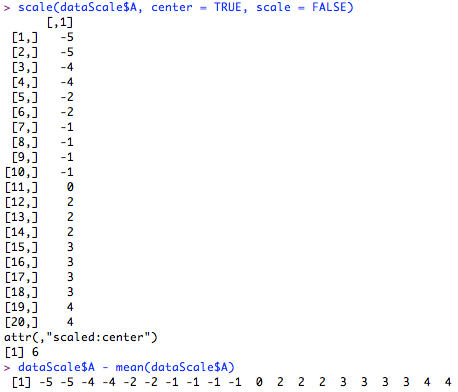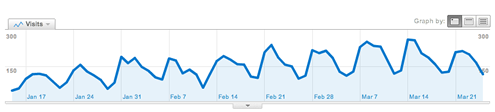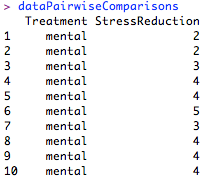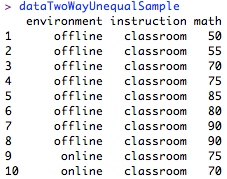# Blog Archives

## Singleton Remover CSV v0.3

April 21, 2014
ByI created a small program called Singleton Remover CSV to help researchers process files quickly that might otherwise take a long time to be handled by hand. The program simply compares ID values given in the first two columns of a .csv file, saves any...

## R Tutorial Series: Centering Variables and Generating Z-Scores with the Scale() Function

March 1, 2012
ByCentering variables and creating z-scores are two common data analysis activities. While they are relatively simple to calculate by hand, R makes these operations extremely easy thanks to the scale() function. Tutorial FilesBefore we begin, you may wan...

## R Tutorial Series: Citing R with EndNote

January 6, 2012
ByUnfortunately, due to the vexing complexities of academic style guides and the limitations of associated software packages, citing a non-standard name, such as Cher, Prince, or R Development Core Team can be problematic. Thankfully, I have discovered a...

## R Tutorial Series: Exploratory Factor Analysis

October 24, 2011
ByExploratory factor analysis (EFA) is a common technique in the social sciences for explaining the variance between several measured variables as a smaller set of latent variables. EFA is often used to consolidate survey data by revealing the groupings ...

## The R Programming Wikibook

July 8, 2011
ByThe R Programming wikibook is an open source community project that "aims to create a cross-disciplinary practical guide to the R programming language." It was launched in June 2011 and is seeking content and contributors. The full call for the R Progr...

## R Tutorial Series: 2011 ANOVA Article Data

March 28, 2011
ByHaving wrapped up a recent flurry of R ANOVA articles (and exhausted my knowledge of the subject), I decided to take a look at the R Tutorial Series' Google Analytics data from the past few months. Since I posted the Two-Way Omnibus ANOVA article on J...

## R Tutorial Series: Applying the Reshape Package to Organize ANOVA Data

March 14, 2011
ByAs demonstrated in the preceding ANOVA tutorials, data organization is central to conducting ANOVA in R. In standard ANOVA, we used the tapply() function to generate a table for a single summary function. In repeated measures ANOVA, we used separate da...

## R Tutorial Series: ANOVA Pairwise Comparison Methods

March 7, 2011
ByWhen we have a statistically significant effect in ANOVA and an independent variable of more than two levels, we typically want to make follow-up comparisons. There are numerous methods for making pairwise comparisons and this tutorial will demonstrate...

## R Tutorial Series: Two-Way ANOVA with Unequal Sample Sizes

February 28, 2011
ByWhen the sample sizes within the levels of our independent variables are not equal, we have to handle our ANOVA differently than in the typical two-way case. This tutorial will demonstrate how to conduct a two-way ANOVA in R when the sample sizes withi...

## R Tutorial Series: Two-Way Repeated Measures ANOVA

February 21, 2011
ByRepeated measures data require a different analysis procedure than our typical two-way ANOVA and subsequently follow a different R process. This tutorial will demonstrate how to conduct two-way repeated measures ANOVA in R using the Anova() function fr...